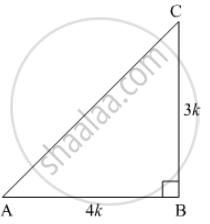# If 4 Tan θ = 3, Evaluate ((4sin Theta - Cos Theta + 1)/(4sin Theta + Cos Theta - 1)) - Mathematics

If 4 tan θ = 3, evaluate ((4sin theta - cos theta + 1)/(4sin theta + cos theta - 1))

#### Solution

Given: 4 tan θ = 3 ⇒ tan θ = 3/4

Let us suppose a right angle triangle ABC right angled at B, with one of the acute angle θ. Let the sides be BC = 3kand AB = 4k, where k is a positive numberBy Pythagoras theorem, we get

AC^2 = BC^2 + AB^2

AC^2 = (3k)^2 + (4k)^2

AC^2 = 9k^2 + 16k^2

AC = sqrt(25k^2)

AC = +- 5k

Ignoring AC = − 5k , as k is a positive number, we get

AC = 5k

if tan theta  = (BC)/(AB) = 3/4 then sin theta = (BC)/(AC) = 3/5 and cos theta = (AB)/(AC) = 4/5

Putting the values in  ((4 sin theta - cos theta + 1)/(4 sin theta +  cos theta - 1)) we get

((4xx3/5 - 4/5 + 1)/(4xx 3/5 + 4/5 -1)) = (((12- 4 + 5)/5)/((12 + 4 - 5)/5)) = 13/11

Concept: Trigonometric Ratios
Is there an error in this question or solution?